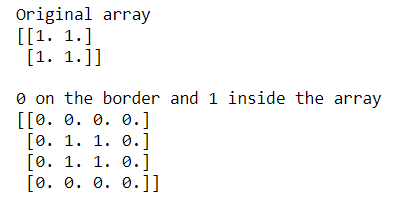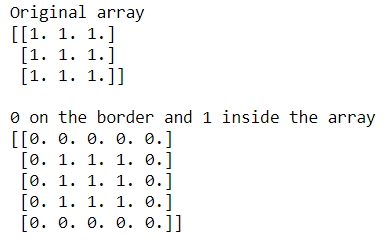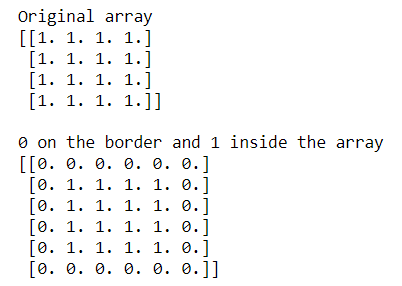Related Articles
How to add a border around a NumPy array?
• Last Updated : 01 Oct, 2020

Sometimes we need to add a border around a NumPy matrix. Numpy provides a function known as ‘numpy.pad()’ to construct the border. The below examples show how to construct a border of ‘0’ around the identity matrix.

Syntax :

```numpy.pad(array, pad_width, mode='constant', **kwargs)
```

Example 1: Construct a border of 0s around 2D identity matrix

## Python3

 `# importing Numpy package``import` `numpy as np`` ` `# Creating a 2X2 Numpy matrix``array ``=` `np.ones((``2``, ``2``))`` ` `print``(``"Original array"``)``print``(array)`` ` `print``(``"\n0 on the border and 1 inside the array"``)`` ` `# constructing border of 0 around 2D identity matrix``# using np.pad()``array ``=` `np.pad(array, pad_width``=``1``, mode``=``'constant'``,``               ``constant_values``=``0``)`` ` `print``(array)`

Output:In the above examples, we construct a border of 0s around the 2-D NumPy matrix.

Example 2: Construct a border of 0s around 3D identity matrix

## Python3

 `# importing Numpy package``import` `numpy as np`` ` `# Creating a 3X3 Numpy matrix``array ``=` `np.ones((``3``, ``3``))`` ` `print``(``"Original array"``)``print``(array)`` ` `print``(``"\n0 on the border and 1 inside the array"``)`` ` `# constructing border of 0 around 3D identity matrix``# using np.pad()``array ``=` `np.pad(array, pad_width``=``1``, mode``=``'constant'``,``               ``constant_values``=``0``)`` ` `print``(array)`

Output:In the above examples, we construct a border of 0s around the 3-D NumPy matrix.

Example 3: Construct a border of 0s around 4D identity matrix

## Python3

 `# importing Numpy package``import` `numpy as np`` ` `# Creating a 4X4 Numpy matrix``array ``=` `np.ones((``4``, ``4``))`` ` `print``(``"Original array"``)``print``(array)`` ` `print``(``"\n0 on the border and 1 inside the array"``)`` ` `# constructing border of 0 around 4D identity matrix``# using np.pad()``array ``=` `np.pad(array, pad_width``=``1``, mode``=``'constant'``,``               ``constant_values``=``0``)`` ` `print``(array)`

Output:In the above examples, we construct a border of 0s around the 4-D NumPy matrix.

Attention geek! Strengthen your foundations with the Python Programming Foundation Course and learn the basics.

To begin with, your interview preparations Enhance your Data Structures concepts with the Python DS Course.

My Personal Notes arrow_drop_up